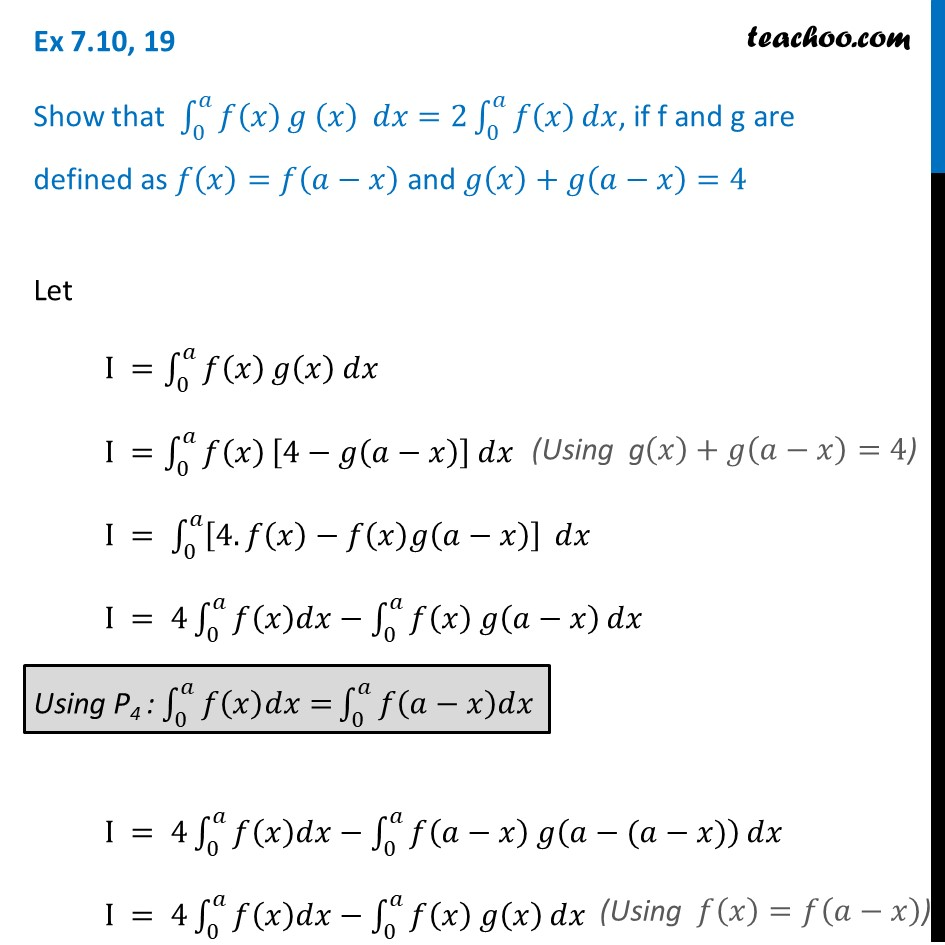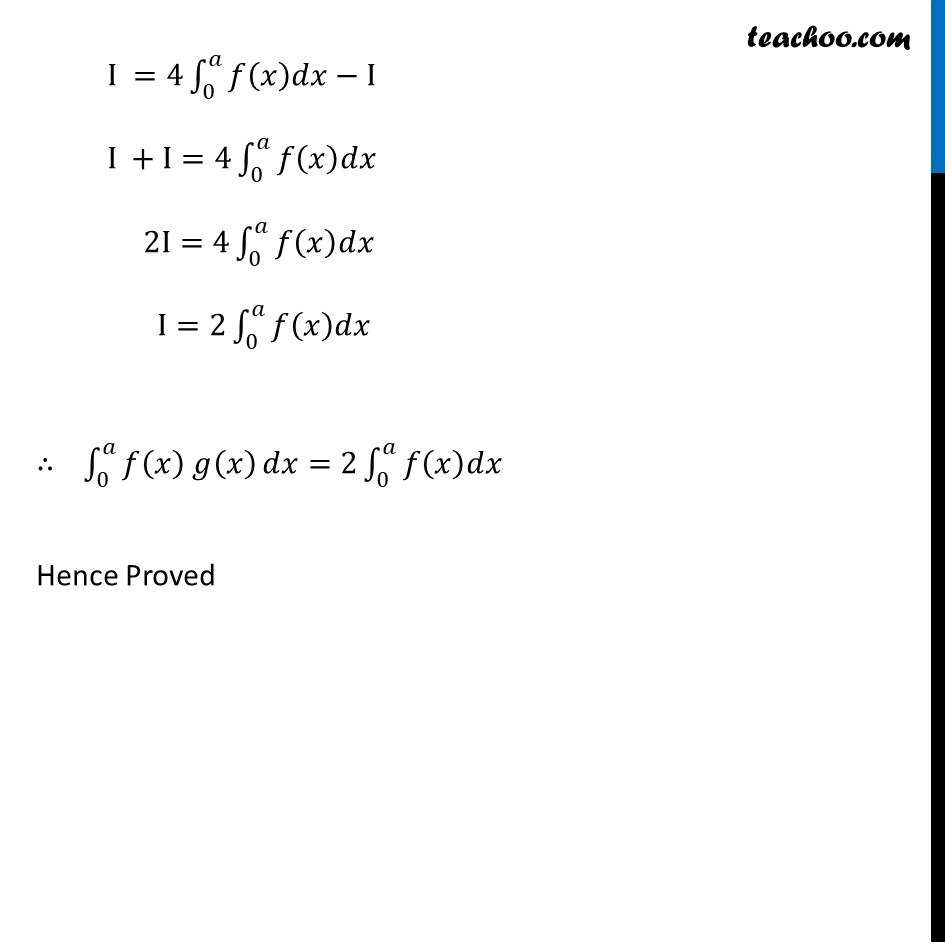Definite Integration by properties - P4

Chapter 7 Class 12 Integrals
Concept wiseLearn in your speed, with individual attention - Teachoo Maths 1-on-1 Class

### Transcript

Ex 7.10, 19 Show that ∫_0^𝑎▒𝑓(𝑥) 𝑔 (𝑥) 𝑑𝑥=2∫_0^𝑎▒𝑓(𝑥) 𝑑𝑥, if f and g are defined as 𝑓(𝑥)=𝑓(𝑎−𝑥) and 𝑔(𝑥)+𝑔(𝑎−𝑥)=4 Let I =∫_0^𝑎▒𝑓(𝑥) 𝑔(𝑥) 𝑑𝑥 I =∫_0^𝑎▒𝑓(𝑥) [4−𝑔(𝑎−𝑥)] 𝑑𝑥 I = ∫_0^𝑎▒[4.𝑓(𝑥)−𝑓(𝑥)𝑔(𝑎−𝑥)] 𝑑𝑥 I = 4∫_0^𝑎▒〖𝑓(𝑥)𝑑𝑥−∫_0^𝑎▒〖𝑓(𝑥) 𝑔(𝑎−𝑥) 〗〗 𝑑𝑥 I = 4∫_0^𝑎▒〖𝑓(𝑥)𝑑𝑥−∫_0^𝑎▒〖𝑓(𝑎−𝑥) 𝑔(𝑎−(𝑎−𝑥)) 〗〗 𝑑𝑥 I = 4∫_0^𝑎▒〖𝑓(𝑥)𝑑𝑥−∫_0^𝑎▒〖𝑓(𝑥) 𝑔(𝑥) 〗〗 𝑑𝑥 I =4∫_0^𝑎▒〖𝑓(𝑥)𝑑𝑥−I〗 I +I=4∫_0^𝑎▒𝑓(𝑥)𝑑𝑥 2I=4∫_0^𝑎▒𝑓(𝑥)𝑑𝑥 I=2∫_0^𝑎▒𝑓(𝑥)𝑑𝑥 ∴ ∫_0^𝑎▒〖𝑓(𝑥) 𝑔(𝑥) 〗 𝑑𝑥=2∫_0^𝑎▒𝑓(𝑥)𝑑𝑥 Hence Proved